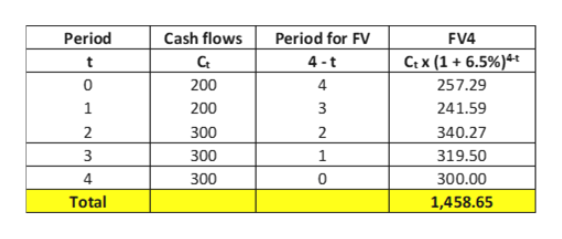# What is the future value of the following set of cash flows 4 years from now? Assume interest rate 6.5%.t      0     1     2     3    4CF 200 200 300 300 300

Question

What is the future value of the following set of cash flows 4 years from now? Assume interest rate 6.5%.
t      0     1     2     3    4
CF 200 200 300 300 300

check_circleExpert Solution
Step 1

Horizon is n = 4 years from now.

FVn = The future value at the end of period n, of a cash flow occuring at the end of period t = Ct x (1 + i)n-t

where Ct = Cash flow occuring at the end of period t and i = interest rate per period.

Step 2

In our case, n = horizon period = 4 years

i = 6.5%

Hence, FV4 = Ct x (1 + 6.5%)4-t

We now proceed to do the calculation in an excel file.

Step 3

Please see the table on the white board. Please be guided by the second row to understand the mathema...help_outlineImage TranscriptionclosePeriod Cash flows Period for FV FV4 Ct x (1+6.5%)4t Ct 4-t t 257.29 0 200 4 1 200 3 241.59 2 2 300 340.27 319.50 3 300 1 4 300 0 300.00 Total 1,458.65 fullscreen

### Want to see the full answer?

See Solution

#### Want to see this answer and more?

Solutions are written by subject experts who are available 24/7. Questions are typically answered within 1 hour*

See Solution
*Response times may vary by subject and question
Tagged in